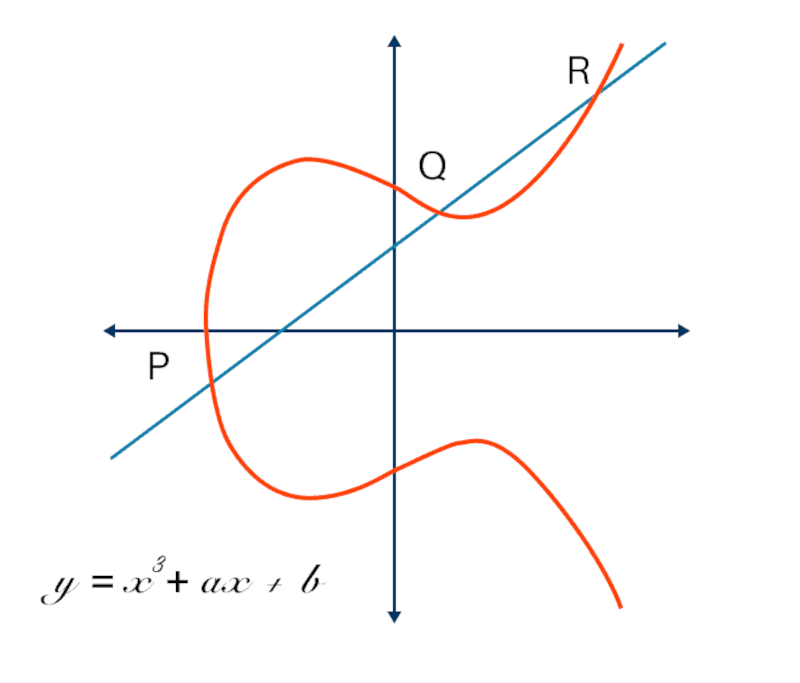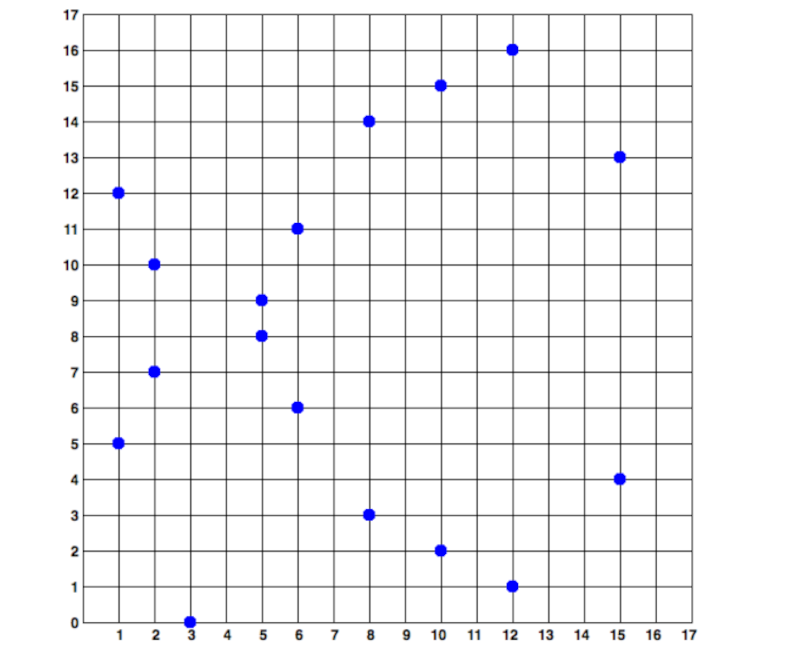# Digital Signatures in BitcoinIn this article, we learn about digital signatures, and how they are created and verified in the Bitcoin protocol.

1. Introduction.
2. Digital Signatures.
3. Creating a Digital Signature.
4. How Digital Signatures are Verified.
5. Summary.

## Introduction

We know that a sender A who wants to transfer funds to sender B has to sign a transaction to prove that they own the private key that corresponds to the public key without having to reveal the private key. It verifies that the sender has the funds he/she intends to send. Just like a bank cheque's signature is used by the sender to authorize a transfer of funds, so are digital signatures in relation to the blockchain. The only exception is that digital signatures used cryptography hashing and algorithms that make it very difficult to forge a signature.

In Bitcoin and Etherium, the ECDSA(Elliptic Curve Digital Signature Algorithm) is used to generate digital signatures. For more on the ECDSA algorithm, we can refer to the links provided in the reference section.

Elliptic Curve Cryptography is a form of public-key cryptography based on the discrete logarithm problem that is expressed by addition and multiplication on the points of the curve.Elliptic curves come in various types, Bitcoin uses the secp256k1 elliptic curve which is defined by the following function.

${\mathrm{y}}^{2}$ mod p = ${\mathrm{x}}^{3}$ + 7 mod p

Above mod p means that the curve is over a finite field of prime order p, hence the following image of a scattered pattern of dots in two dimensions.The above elliptic curve is over a finite field of the prime order 17. On the other hand, the secp256k1 bitcoin curve would be a complex pattern of such dots on a very large grid.

By using this algorithm we can use a private key to derive a public key by not a public key to derive the private key. This is referred to as a trap door function since the elliptic curve mathematics works in one direction. This makes transactions secure and tamper-proof in the blockchain.

The ECDSA algorithm is implemented using the script programming language functions such as;

• OP_CHECKSIG - used to verify that the digital signature used in a transaction input is valid. Returns True or False.
• OP_CHECKSIGVERIFY - executed after the former for verification purposes. Returns nothing if it succeeds otherwise an error.
• OP_CHECKMULTISIG - compares a digital signature against the public key until a match is found. It returns True or False.
• OP_CHECKMULTISIGVERIFY - Similar to the former but executed after it. It returns nothing if successful.

## Digital Signatures.

A digital signature is a mathematical scheme that is used to prove the authenticity of a digitally signed message, in this case, it does this for a transaction in the blockchain. A digitally signed message not only proves authenticity but also proves the non-repudiation and integrity of the message.

In Bitcoin a digital signature has three functions namely;

• To prove ownership of funds by the owner of the private key intends to send to a recipient.
• To prove the above authorization is valid and non-repudiable.
• The transaction can't be mutated after it is signed.

Digitally signing transactions on the blockchain consists of an algorithm used to create a signature using the private key from a transaction and an algorithm that allows anyone(peers) to verify it.

## Creating a Digital Signature.

We use the following function to create digital signatures;

Sig = Fsig(Fhash(m),dA)

Where;

• Sig - the resulting signature.
• Fsig - the signing algorithm.
• Fhash - the hashing function.
• m - the message, in this case a transaction.
• dA - private key used in the signing.

The function can be summarized as follows;
Fsig generates a temporary key pair comprised of public and private keys. This pair is used to calculate R and S after the transformation involving the signing of the private key Fsig(.., dA) and the transaction hash (Fhash(m))
The temporary pair is based on a random number k. k is also used as a temporary private key, it is also used in the derivation of a corresponding temporary public key P (P = K*G).
R in the digital signature is the x coordinate of the temporary public key P.
Now S = k^-1(Hash(m) + dA * R) mod p.
Above;

• k = temporary private key
• R = x-coordinate of the temporary public key
• p - the prime order.

When R and S have been calculated, they are serialized into a byte stream. This byte stream is then encoded using DER.

DER(Distinguished Encoding Rules) is secure encryption that guarantees that a digital signature can be used in any environment such as different types of wallets or devices. This is achieved by making its resolution deterministic from the start to the end. In Bitcoin, it guarantees that the digital signatures are secure under all circumstances.

Consider the following signature which consists of a byte stream of R and S values;

``````3045022100884d142d86652a3f47ba4746ec719bbfbd040a570b1deccbb6498c75c4ae24cb02204b9f039ff08df09cbe9f6addac960298cad530a863ea8f53982c09db8f6e381301
``````

Once we break it down and have the following components;
0x30 - start of the encoding sequence
0x45 - sequence length
*0x02 - integer value
0x21 - integer length
R - 00884d142d86652a3f47ba4746ec719bbfbd040a570b1deccbb6498c75c4ae24cb
0x02 - another integer
0x20 - integer length
0x01 - type of signature hash used by the signature.

Above, the values R and S are the important ones as we will use them to verify this signature.

In Summary the steps of creating a digital signature consist of two major parts;

1. The first involves generating a random number which is multiplied by the generator point on the curve. We take the x-coordinate(half of the digital signature) of the generated point. This is R.
2. The second part involves taking the private key and multiplying it with R([R * private_key]). We then include the message we intend to sign. i.e ([R * private_key] + message). The result of this is the signature - S itself. Now we can use R and S to prove that we own the private key for the corresponding public key.

## How Digital Signatures are Verified.

Digital signature verification requires the digital signature (R, S), the serialized transaction and the public key corresponding to the private key used to create the signature.
We say that a digital signature is valid if the owner of the private key used to generate the public key also produced this signature on this transaction.

A verification algorithm takes in a message which is then hashed transaction, the signer's public key, and the signature(R and S). The output should be True if the signature is valid, and False otherwise.

Since verification is the inverse of the generation function, R and S together with the public key are used to calculate P - a point on the elliptic curve.
We have the following;
P = S^-1 * Hash(m) * G + S^-1 * R * Qa, where;

• R, S = signature value.
• Qa = the recepients public key.
• m = the transaction.
• G = the elliptic curve generator.

If the x-coordinate of P is equal to R, the signature is considered valid, otherwise, it is invalid.

In Summary the steps of verifying a digital signature consist of finding three major points on the elliptic curve;

Remember our goal here is to verify that the used public key and digital signature were created using the same private key. In this case, the recipient uses the discussed parts in the previous section to find two new points on the curve.

1. To obtain the first point, we divide the message by S i.e (message / S). This point is just the generator point multiplied by this value -> (message / S).
2. To obtain the second point we divide R and S i.e (R / S). This point is just the public key multiplied by this value -> (R / S).
3. Finally to obtain the third point on the curve, we add the above two points. In this case, if the x-coordinate of the third point is similar to the x-coordinate of the random point r that we began with, then it is proof that the digital signature was created using the private key corresponding to this public key.